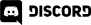# Home > Programming >

24. November 2012

It is quite common to want to rotate one point relative to another the location of another point.  This math recipe looks at exactly this process.

## Just the math

angle = (angle ) * (Math.PI/180); // Convert to radians

var rotatedX = Math.cos(angle) * (point.x - center.x) - Math.sin(angle) * (point.y-center.y) + center.x;

var rotatedY = Math.sin(angle) * (point.x - center.x) + Math.cos(angle) * (point.y - center.y) + center.y;

return new createjs.Point(rotatedX,rotatedY);

## Description

The angle conversion is entirely dependant on your math libraries.  In the case of JavaScript's Math library ( and the standard C++ libraries ), cos and sign expect the angles to be expressed in radians.  As you can see, the conversion formula is quit simple.

As to the math, the rotated location of the X value is found by taking the cos of the angle to rotate by, multiplied by the distance between the X value of the point you want to rotate and the point to rotate around minus the sin of the angle multiplied by the distance between the points, then finally add the x location of the point.  The calculation for rotating in the Y direction is basically identical, except the sin and cos calculations are swapped and you subtract instead of adding.

One thing to keep in mind here is the rotation is relative to the current position not the total rotation.  For example, if you are currently at 90 degrees and want to rotate to 135 degrees, you would use an angle of 45, not 135.

## Complete code

<!DOCTYPE html>

<html>

<script src="http://code.createjs.com/easeljs-0.5.0.min.js"></script>

<script>

var ball1,ball2;

var stage;

function rotatePoint(point, center, angle){

angle = (angle ) * (Math.PI/180); // Convert to radians

var rotatedX = Math.cos(angle) * (point.x - center.x) - Math.sin(angle) * (point.y-center.y) + center.x;

var rotatedY = Math.sin(angle) * (point.x - center.x) + Math.cos(angle) * (point.y - center.y) + center.y;

return new createjs.Point(rotatedX,rotatedY);

}

function demo(){

stage = new createjs.Stage("theCanvas");

var g = new createjs.Graphics();

g.setStrokeStyle(1);

g.beginFill(createjs.Graphics.getRGB(0,0,255));

g.drawCircle(0,0,30);

ball1 = new createjs.Shape(g);

ball2 = new createjs.Shape(g);

ball1.x = stage.canvas.width/2;

ball1.y = stage.canvas.height/2;

ball2.x = stage.canvas.width/2;

ball2.y = 30;

//And go...

stage.update();

// onFrame will be called each "tick". Default is 50ms, or 20FPS

}

function onFrame(elapsedTime) {

// Convert from milliseconds to fraction of a second

var delta = elapsedTime /1000;

// Rotate by 90 degrees per second, or 1 full rotation per 4 seconds.

var rotateBy = 90 * delta;

// Current position of ball2

var ballPosition = new createjs.Point(ball2.x,ball2.y);

// Updated position rotated by... um... rotateBy value

var rotatedPosition = rotatePoint(ballPosition,

new createjs.Point(ball1.x,ball1.y),

rotateBy);

// Update ball's position to the newly rotated coordinates

ball2.x = rotatedPosition.x;

ball2.y = rotatedPosition.y;

stage.update();

}

</script>